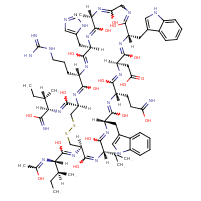Structure InformationCompound Identification

SMILES

CC[C@H](C)[C@@H](NC(=O)[C@@H]1CSSC[C@H](NC(=O)[C@@H](NC(C)=O)[C@@H](C)CC)C(=O)N[C@@H](C(C)C)C(=O)N[C@@H](CC2=CNC3=CC=CC=C23)C(=O)N[C@@H](CCC(N)=O)C(=O)N[C@H](CC(O)=O)C(=O)N[C@@H](CC2=CNC3=CC=CC=C23)C(=O)NCC(=O)N[C@@H](C)C(=O)N[C@H](CC2=CNC=N2)C(=O)N[C@@H](CCCN=C(N)N)C(=O)N1)C(N)=O

InChIKey

InChIKey=PFDGVPWHMGJHFE-KBNXDDINSA-N

Formula

C73H104N22O17S2

Mass

1625.89

### Export to:

Taxonomic Classification

Taxonomy Tree

Kingdom

Organic compounds

Superclass

Organic Polymers

Class

Polypeptides

Subclass

Not available

Intermediate Tree Nodes

Not available

Direct Parent

Polypeptides

Alternative Parents

Molecular Framework

Aromatic heteropolycyclic compounds

Substituents

Polypeptide - Cyclic alpha peptide - Isoleucine or derivatives - Macrolactam - N-acyl-alpha amino acid or derivatives - Alpha-amino acid amide - N-substituted-alpha-amino acid - Alpha-amino acid or derivatives - 3-alkylindole - Indole or derivatives - Indole - Imidazolyl carboxylic acid derivative - Benzenoid - Fatty amide - Fatty acyl - Substituted pyrrole - N-acyl-amine - Imidazole - Pyrrole - Acetamide - Heteroaromatic compound - Azole - Secondary carboxylic acid amide - Primary carboxylic acid amide - Lactam - Organic disulfide - Carboxamide group - Guanidine - Azacycle - Carboxylic acid derivative - Carboxylic acid - Organoheterocyclic compound - Organic 1,3-dipolar compound - Propargyl-type 1,3-dipolar organic compound - Carboximidamide - Monocarboxylic acid or derivatives - Organic oxide - Hydrocarbon derivative - Carbonyl group - Organic nitrogen compound - Organooxygen compound - Organonitrogen compound - Organic oxygen compound - Aromatic heteropolycyclic compound

Description

This compound belongs to the class of organic compounds known as polypeptides. These are peptides containing ten or more amino acid residues.

External Descriptors

Not available